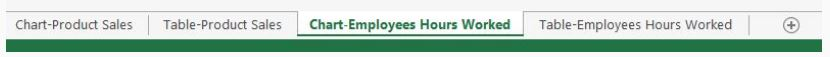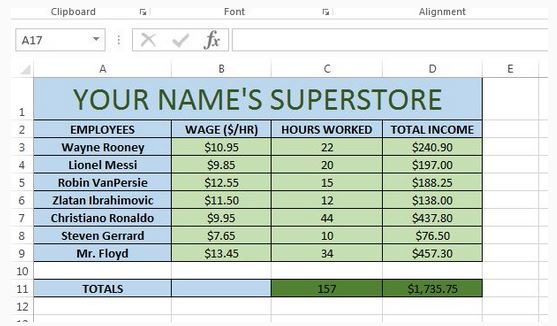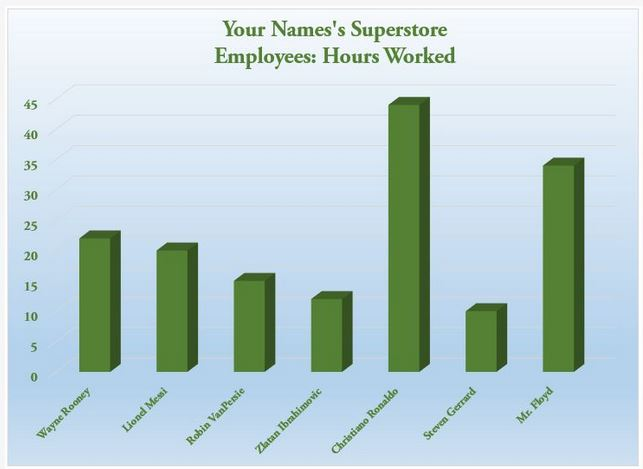# MSExcel - Adding Another Table and Chart

You should open up the spreadsheet that you've been working on and then add a new sheet.
By the end of this task you will have four sheets that are names as follows:The new table that you create should resemble the following:Note that...
Cell D3 to D9 should be a formula where you multiply the hours worked by the wage for each employee.
Also, cells C11 and D11 are formulas that sum up the data in the column.

Once you have completed the table you will add the Hours Worked information to a chart. The chart should resemble the following: